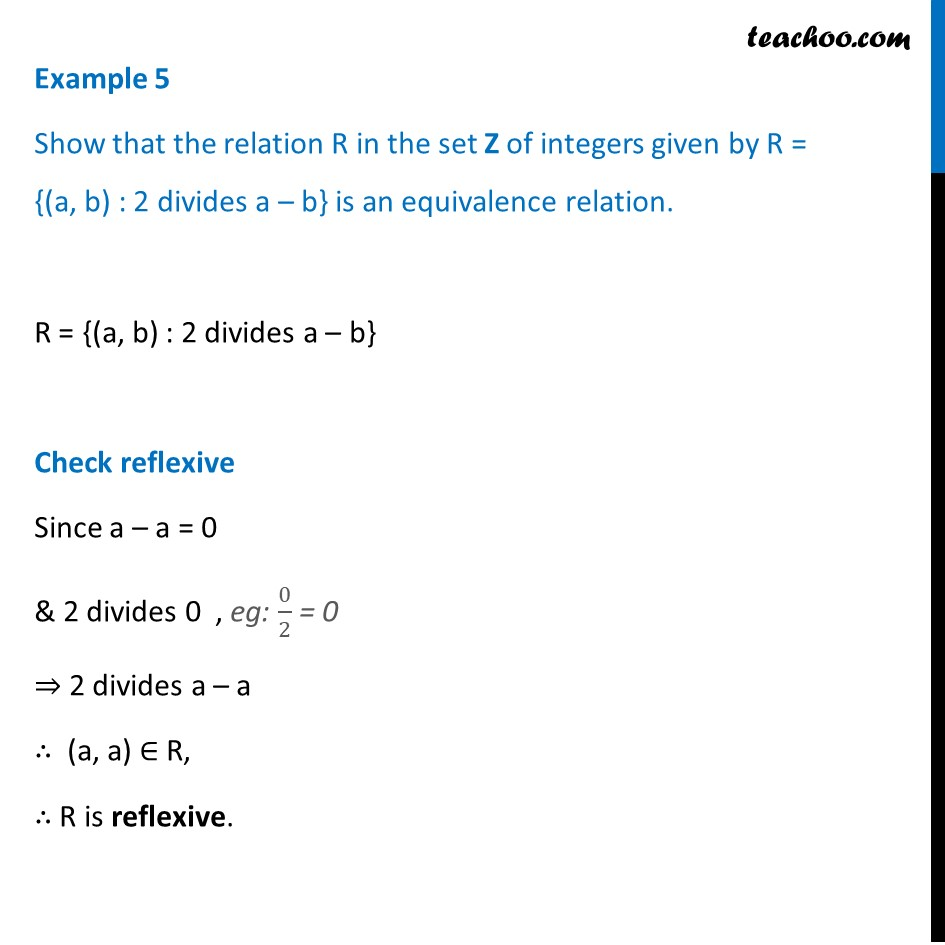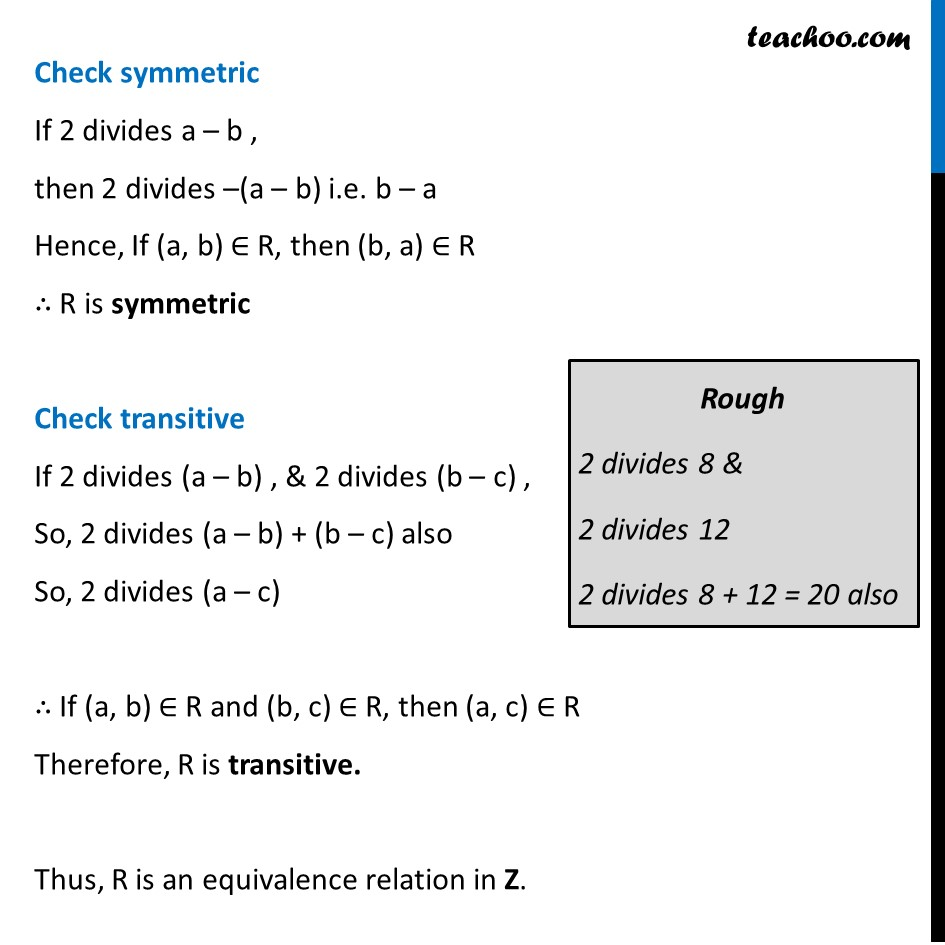Examples

Chapter 1 Class 12 Relation and Functions
Serial order wiseLearn in your speed, with individual attention - Teachoo Maths 1-on-1 Class

### Transcript

Example 5 Show that the relation R in the set Z of integers given by R = {(a, b) : 2 divides a – b} is an equivalence relation. R = {(a, b) : 2 divides a – b} Check reflexive Since a – a = 0 & 2 divides 0 , eg: 0/2 = 0 ⇒ 2 divides a – a ∴ (a, a) ∈ R, ∴ R is reflexive. Check symmetric If 2 divides a – b , then 2 divides –(a – b) i.e. b – a Hence, If (a, b) ∈ R, then (b, a) ∈ R ∴ R is symmetric Check transitive If 2 divides (a – b) , & 2 divides (b – c) , So, 2 divides (a – b) + (b – c) also So, 2 divides (a – c) ∴ If (a, b) ∈ R and (b, c) ∈ R, then (a, c) ∈ R Therefore, R is transitive. Thus, R is an equivalence relation in Z.